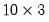Command Reference : Matrix Language Reference

 @vec
Vectorize (stack columns of) matrix.
Syntax: @vec(m)
m: matrix, sym
Return: vector
Creates a vector from the columns of the given matrix stacked one on top of each other. The vector will have the same number of elements as the source matrix.
Examples
matrix m1 = @mrnd(10, 3)
vector v1 = @vec(m1)
creates amatrix of uniform random numbers M1 and stacks it into a 30 element vector V1.
Cross-references